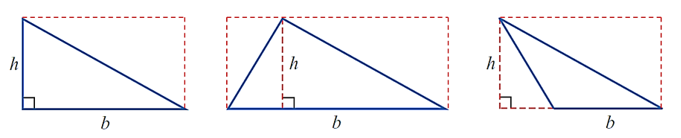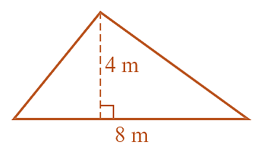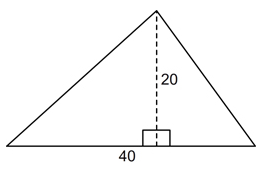PRIMARY

2 - 6

SECONDARY

7 - 10

SENIOR

11 - 12

Home

# Area of a Triangle

Theory & Practice Questions.

## Lesson

#### Area of a Triangle

Area of a triangle = 1/2 base of the triangle x the perpendicular height

#### [Formula]

 A = 1/2 bh## Example

Find the area of the triangle.## Explanation

Area of a triangle = 1/2 base of the triangle x the perpendicular height

A = 1/2 bh

Substitute given values into the formula

A = 1/2 x 8 x 4

A = 16 m²

## Question

Find the area of the following. Lengths are given in millimetres.## Solution

Area of a triangle = 1/2 x base x ⊥

A = 1/2 x 40 x 20

A = 1/2 x 800

A = 400 mm²